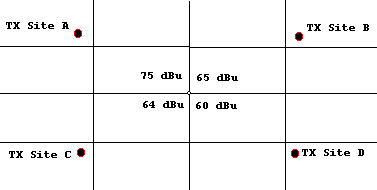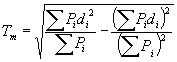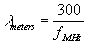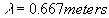# How is “composite” or “aggregate” coverage computed in TAP?

#### The TAP Composite Coverage Program

The TAP Composite Coverage Program combines the results of two or more tile studies into one study that you can view using the TAP Tile Threshold plotting program.

In the process of combining files, TAP will perform specific operations on selected tile field strength coverage or shadow studies. The contents of the resulting tile study file will differ from the input files in some pre-defined manner. Math operations to generate Composite coverage values will operate on overlapping tile or radial study regions.

This program would allow you to evaluate coverage over a tile area from different transmitter sites, or to evaluate the coverage from a single site with different facilities (tower heights, antenna patterns, etc.).  After the studies have been selected, you will direct TAP to combine your input files using one of several Mathematical Operations:

• Maximum: Write the greatest value at each point in your tile region to the results file.
• Best Server: As discussed below, write a value at each tile point only if this value meets a pre-defined “Best Server” selection criteria.
• Simulcast (Delay Spread): As discussed below, write a value at each tile point only if this value meets a pre-defined “Simulcast” selection criteria based on signal strength and the computed Simulcast Delay Spread value.

#### Best Server

The Best Server function evaluates the value from each input study at each tile point. The maximum coverage value at each tile point would be written to the results file ONLY IF the differences between this value and all other computed coverage values at the same location are greater than or equal to a user-defined value (a “capture margin”). In this manner, you will build a condition of sufficient isolation between signals at each field strength point on the tile. Any point without the specified separation between the signal levels would be considered a point of no service, regardless of the magnitude of the highest signal.

As an example, suppose you wish to generate a best server study from four input field strength tile studies. Assume further that you have specified a desired capture margin of 10 dB. In the picture below, the 75 dBu field strength value at the center of the tile region will be written to the results file because it exceeds the next highest value (65 dBu) by 10dB.On the other hand, if you had specified a capture margin of 15 dBu, a “dummy value” would be written to the results file indicating that the best server conditions were not met. You will then assign a particular color or fill pattern to each point that meets the best server criteria and view these points in a TAP Threshold plot.

The combined effect of multiple simulcast signals can also be computed using the “Delay Spread” factor.  This factor is given in Handbook of Land-Mobile Radio System Coverage (Garry C. Hess, Artech House, 1998, p. 131) as a function of the power P and the delay d (based on distance) for each received signal:In TAP, when you select the Simulcast (Delay Spread) type of Composite Coverage, you must enter two parameters, the Capture Margin and the Maximum Simulcast Delay Spread (SDS) value.  The “Capture Margin” is the value described under “Best Server” above. If any one signal exceeds all others by this value, then this single signal is sufficient to be recognized by the receiver, and the point in question is assigned this value.  If the capture margin criterion is not met, the spread value is computed from the equation from Hess above. The “Maximum SDS” is the maximum permitted delay value. If the computed delay spread exceeds the maximum value, then the point is considered to have no usable signal. If the computed delay is less than the maximum specified delay, the signal at the point is the computed sum of the received power of the signals (added linearly).

#### Separate Transmitter Phasing Values

In the SDS study, you can also assign a relative phase value (in degrees) or a “launch delay” (in microseconds) to each of the transmitters. This value would be a hardware-related variable that represents the total phase value for each transmitter, including factors such as transmission line lengths and electronically adjusted phasing.  As an example, consider two transmitters, A and B, with the following parameters relative to a receive location under consideration:

Transmitter A Transmitter B
Frequency (always the same for a simulcast system) 450 MHz 450 MHz
Computed field at RX 55 dBu 49 dBu
Distance to RX 11 km 15 km
Launch Delay +15° (+0.04166λ) -30° (-0.0833λ)

We can arbitrarily designate Transmitter A as the reference. (Normally the phasing of the reference would be zero, but for this illustration use the values shown above.)
The wavelength in meters is:Computing the distance from each transmitter to the receive location in wavelengths:
Transmitter A: 11km /.667meters = 16,491.75412λ
Transmitter B: 15km /.667meters = 22,488.75562λ

Since Transmitter A is the reference, we need the difference in phase between that reference and the arriving signal from Transmitter B. This difference is computed considering both the signal phasing at the transmitter and the phasing effect of the distance to the receive site.  The difference between phases of the arriving signals is the phase of B (launch delay for “B” plus distance from “B” transmitter) minus the phase of A (launch delay for “A” plus distance from “A” transmitter) :
Δψ = (-.0833λ + 22,488.75562λ ) – (.04166λ + 16,491.75412λ )
Δψ = 5996.876539λ

We only need be concerned about the non-integer portion of wavelength differences. (Signals with phase differences that are an integer number of wavelengths would be perfectly in phase.) Considering the fractional wavelength difference:
Δψ = .876539λ • 360°/λ
Δψ = 315.55404λ

The composite field is the sum of the reference value, plus each other component added as a vector considering the phase difference. First, the computed field strength magnitude of each component must be converted to μ V/m so the values can be added:
Transmitter A: 55dBu = 20 Log (E) => Ea = 562.34 μV/m
Transmitter B: 49dBu = 20 Log (E) => Eb = 281.84 μV/m

The composite signal is:
Ea + Eb• Cos(Δψ)
562.34 + 281.84• Cos(315.55404°) = 763.549 μV/m = 57.66 dBu